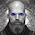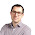## Saturday, August 10, 2013

### You know what really grinds my gears? (In Python)

I can never correctly remember when things are passed as references or copied as local variables inside functions.

Take these two, innocuously looking functions. Because both do the same thing (namely set the contents of a vector, P, to [1, 1]) I call them 1 and a, respectively, since one is not better than the other.

def implementation_1(P):

P = [1, 1]

def implementation_a(P):

P = 1
P = 1

What you would expect is one of the following two options
1. Both functions change P to [1, 1] (permanently).
2. Both functions take a local copy of P and change it to [1, 1], and after the function returns, the local [1, 1] array is forgotten.

A simple test is to do this:

P = [0, 0]
print P

implementation_1(P)
print P

implementation_a(P)
print P

which prints:

[0, 0]
[0, 0]
[1, 1]

So clearly implementation_a() is different from implementation_1(), although they seemingly do the same.

1.Another curiosity is this suggestion (By Jimmy Kromann):

def implementation_I(P):

P = P + list()

P = 1
P = 1

which does the same thing as implementation_1().

2.while

def implementation_alpha(P):

P = P

P = 1
P = 1

works like implementation_a()

3.It's something to do with Python using names, rather than pointers, and the semantics are different.

In implementation 1, the name "P" is assigned to the original list [1, 1]. It is then assigned to a new local list [0, 0]. Assigning "P" to different things has no effect on the original list.

In implementation 2, the name "P" is assigned to the original list [1, 1]. Then the contents of the original list are altered, via P=1 etc.

4.Yeah ... I guess you can say the difference is in one case you are using the [] operator on P and in the other you are using the = operator on P, and they work differently.

You can print out id(P) and you'll see different addresses (or ids) after using the = operator.

5.If you really want to confuse yourself, use an empty list as the default value for a parameter in a function:

>>> def A(param=[]):
... param.append(1)
... print param
...
>>> A([1,2,3]) # Works as expected
[1, 2, 3, 1]
>>> A([4,5,6]) # Works as expected
[4, 5, 6, 1]
>>> A() # Works as expected

>>> A() # Hmmm?
[1, 1]
>>> A() # The what??
[1, 1, 1]
>>>

(never use an empty list in a function specification)

6.@Noel O'Boyle: Holy Mother of God!